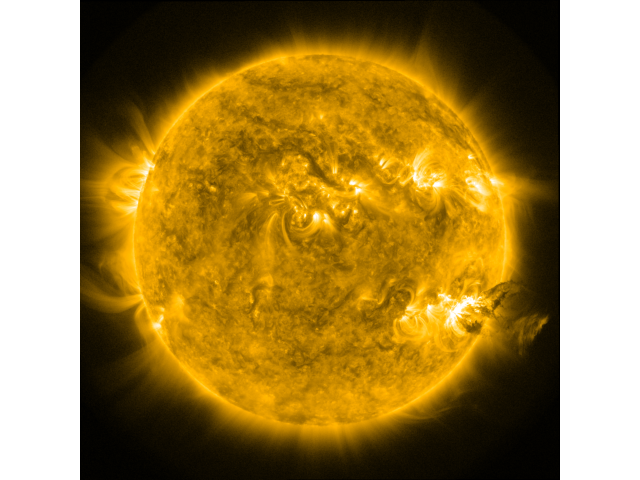# Plotting a Map without any Axes¶

This examples shows you how to plot a Map without any annotations at all, i.e. to save as an image.

```import matplotlib.pyplot as plt
import numpy as np

import sunpy.map
from sunpy.data.sample import AIA_171_IMAGE
```

Create a sunpy map from the sample data.

```smap = sunpy.map.Map(AIA_171_IMAGE)
```

Plot the Map without a frame. We can setup a frameless figure and an axes which spans the whole canvas.

```figure = plt.figure(frameon=False)
ax = plt.axes([0, 0, 1, 1])
# Disable the axis
ax.set_axis_off()

# Plot the map. Since are not interested in the exact map coordinates, we can
# simply use :meth:`~matplotlib.Axes.imshow`.
norm = smap.plot_settings['norm']
norm.vmin, norm.vmax = np.percentile(smap.data, [1, 99.9])
ax.imshow(smap.data,
norm=norm,
cmap=smap.plot_settings['cmap'],
origin="lower")
```
```<matplotlib.image.AxesImage object at 0x7fe204713310>
```

At this point you could save the figure with `plt.savefig()` or show it:

```plt.show()
```Total running time of the script: ( 0 minutes 0.405 seconds)

Gallery generated by Sphinx-Gallery